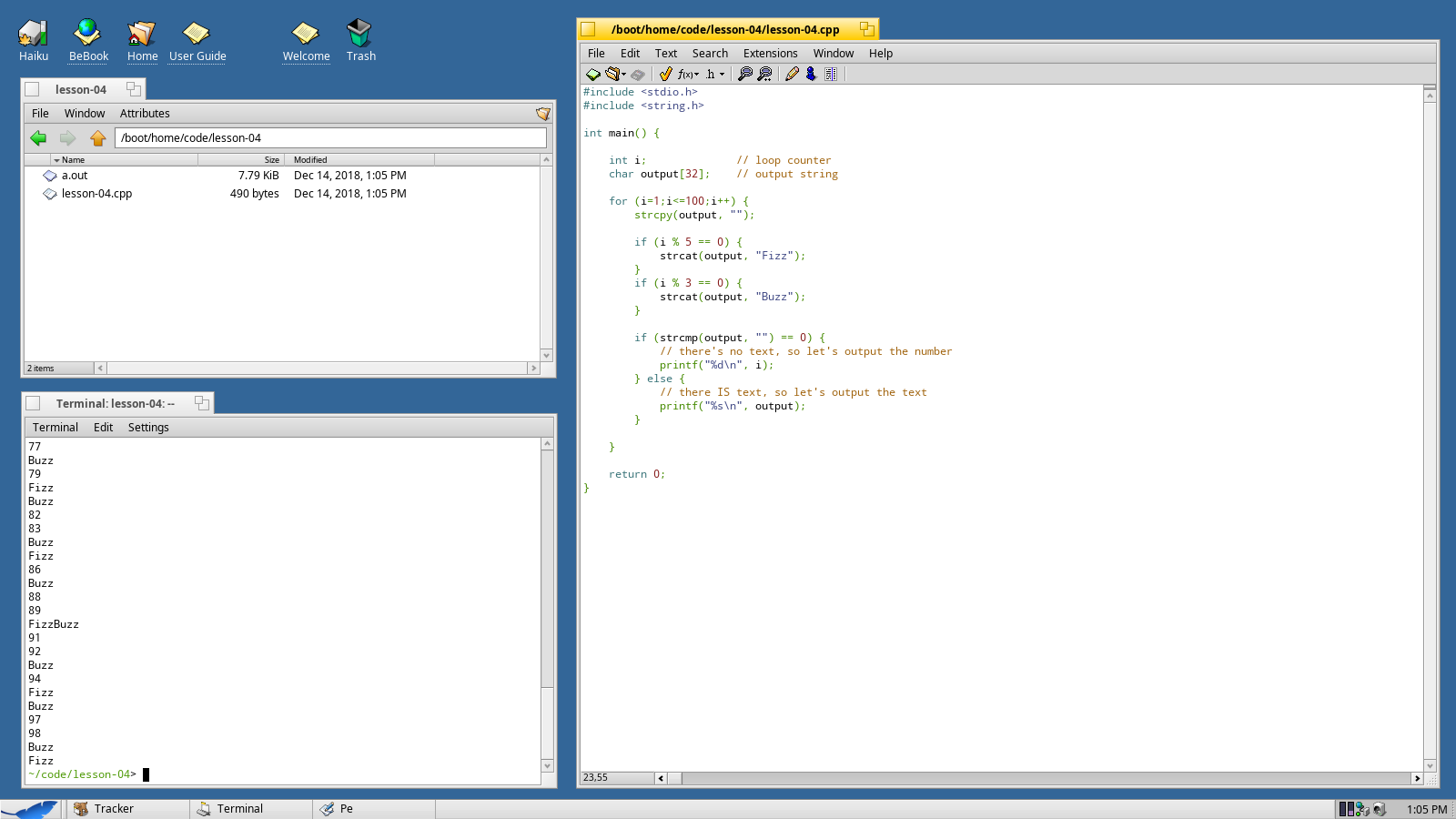Web Developer

# Lesson 4 - FizzBuzz

FizzBuzz is a simple counting game. You count up from 1 and for every 5th number you say "fizz," for every 3rd number you "buzz," and if it's both you say "fizz buzz."

In this lesson we're going to write a program that plays FizzBuzz. You'll learn about the incredibly important `if` and `else` statements, as well as some basic functions for working with text.

Before I get started, I should point out that there are dozens of ways a program could play FizzBuzz and I've chosen to use a method described by YouTuber Tom Scott.

## Set Up a Basic Loop

If you've been following along we'll skip ahead a bit. We're going to start off with a very basic `main` function and a loop that runs from 1 to 100.

Note that the loop has changed - `i=1` and `i<=100`. We aren't dealing with an array this time. We want to make sure we start at 1 and that we include 100.

We're also going to be using the library `string.h`.

``````#include <stdio.h>
#include <string.h>

int main() {

int i;            // loop counter

for (i=1; i<=100; i++) {

}

return 0;
}``````

## Introduction to Strings

It's time to introduce you to a new data type, `char`. A `char` stores one character - a letter, number, spaces, punctuation, etc… If you string enough of them together, you can make words or paragraphs.

In C, a string is a sort of informal data type for holding text - but what you're really working with is an array of characters. Let's define a new string to hold our output:

``````int i;            // loop counter
char output;    // output string``````

An important thing to understand about strings is that you can't simply use `=` to assign them a value. Let's place the following code inside our loop so that we can clear our output each time around.

``strcpy(output, "");``

This will copy the string literal "" into our array named `output`.

## If Statements

Now we need to see if the current number `i` is divisible by 5, divisible by 3, by both, or by neither. Any number that's divisible by 5 will, of course, have a remainder of 0 - so we're going to use the modulus operator `%` again to check.

``````if (i % 5 == 0) {

}
if (i % 3 == 0) {

}``````

And just when you though I couldn't throw another curveball at you, note the double equal sign `==`. In C/C++ a single equal sign is for assignment - copying a value into a variable. The double equal sign is used for comparison - checking to see if two things are equal.

I know it sounds crazy, but there's a really good reason for this that we'll touch on in a future lesson.

## String Concatenation

It's time to learn a great little vocabulary word, concatenation. If you want to combine one string with another, you can't simply add them. (Remember, these are just arrays of characters - adding an array to an array doesn't make any sense.) Instead we need to use a function to concatenate the strings together.

``````if (i % 5 == 0) {
// put "Fizz" at the end of the output
strcat(output, "Fizz");
}
if (i % 3 == 0) {
// put "Buzz" at the end of the output
strcat(output, "Buzz");
}``````

Remember that we don't start with literally nothing, we start with an empty string. If the number is divisible by 5, we put "Fizz" at the end of our empty string and get "Fizz." If the number is also divisible by 3, we put "Buzz" at the end of "Fizz" and get "FizzBuzz."

## If and Else

It's time now to handle our output. Remember the original problem - if it's divisible by 5 or 3 or both we're outputting text. If not, we're outputting the number. We can set this up using `if`, `else`, and a new function `strcmp()` for comparing strings.

``````if (strcmp(output, "")==0) {
// there's no text, so let's output the number
printf("%d\n", i);
} else {
// there IS text, so let's output the text
printf("%s\n, output);
}``````

## Putting it All Together

You can view the full source code below.

``````#include <stdio.h>
#include <string.h>

int main() {

int i;            // loop counter
char output;    // output string

for (i=1; i<=100; i++) {
strcpy(output, "");

if (i % 5 == 0) {
// put "Fizz" at the end of the output
strcat(output, "Fizz");
}
if (i % 3 == 0) {
// put "Buzz" at the end of the output
strcat(output, "Buzz");
}

if (strcmp(output, "")==0) {
// there's no text, so let's output the number
printf("%d\n", i);
} else {
// there IS text, so let's output the text
printf("%s\n, output);
}
}

return 0;
}``````

And you can see it in action below.In this lesson you've learned a bit about dealing with strings of text, including `char`, `strcpy`, `strcat` and `strcmp`. You've also learned how to compare values and make decisions using `if` and `else`.

The `if`, `else` and `for` tools are some of the most frequently used tools in C/C++ and you'll be getting loads of practice with them.